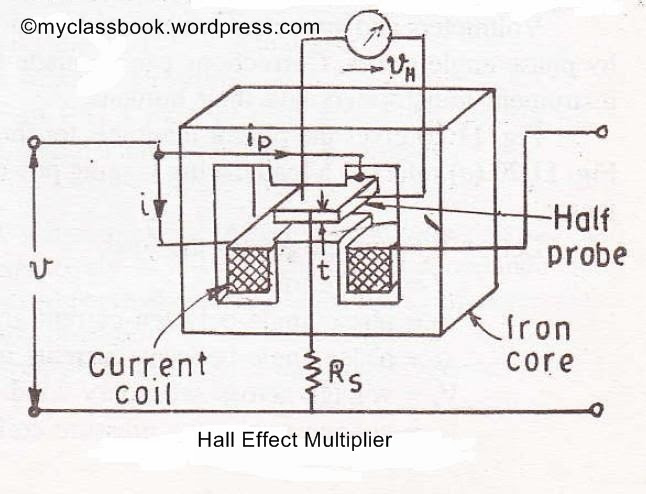# Hall Effect Multiplier - Construction, Working Principle and Applications

Hi friends, in this article we are going to learn Hall Effect Multiplier which can be used in applications where power need to be controlled. Hall Effect Multiplier is also used where the multiplication of signals over a wide range of amplitudes is performed. Now let us see the construction and working of Hall Effect Multiplier.

### Hall Effect:

"When a current carrying conductor is placed in a magnetic field, a transverse effect is noted. This effect is called Hall Effect." It was discovered by scientist Hall in 1879.

Hall found that: “When a magnetic field is applied at right angles to the direction of electric currents, an electric field is setup which is perpendicular to both the direction of electric current and the applied magnetic field.

In other words: “When any specimen carrying a current 'I' is placed in the transverse magnetic field B, then an electric field 'E' is induced in the specimen in the direction perpendicular to both 'I' and 'B'. The phenomenon is known as Hall effect.

### Applications of Hall Effect Multiplier:

1. Determining whether a semiconductor is N-type or P-type.

2. Determining the carrier concentration.

3. Calculating the mobility, having measured the conductivity.

4. Magnetic field meter: the hall voltage vH for a given current is proportional to B. hence the measurement of vH measure the magnetic field B.

5. Hall Effect multiplier: the instrument gives an output proportional to the product of two signals. Thus if the current I made proportional to one input and if B is proportional to the second input, then Hall voltage vH is proportional to the product of two signals.

### Hall Effect Multiplier:

In applications where the power is to be controlled or processed further Hall Effect Multipliers are used. A Hall Effect multiplier is shown in the figure. The Hall Effect multiplier uses a Hall Effect element.

[caption id="" align="alignnone" width="646"]Hall Effect Multiplier[/caption]

The current is passed through the current coil which produces a magnetic field proportional to the current I .this field is perpendicular to the Hall Effect element. A current Ip, proportional to the voltage, is passed through the Hall Effect element in a direction perpendicular to field as shown. The current is limited by the multiplier resistance Rs. The output voltage of the Hall Effect multiplier is:

vH = (KH Ip B) / t

Where,

• KH = Hall co-efficient = V- m/A - Wb m-2

• B = flux density = Wb/m2

• t = thickness of hall element = m

Now B ∝ Vi and ip = v/Rs ∝ v

Hence, vH ∝ Vi

Therefore the output voltage of the Hall Effect Multiplier is proportional to instantaneous power.

Hence the voltmeter connected at the output terminals can be calibrated in terms of power. The Hall Effect voltage which is of the power can be processed further for control and other purposes. This is the major advantage of Hall Effect multiplier over electrodynamometer wattmeters the output of the later being the deflection of a pointer which cannot be processed further.

I hope you understood the construction and working of Hall Effect Multiplier and its Applications. If you have any doubts please let us know. Share your doubts in the comments below. If you liked this article please share and like our facebook page. Have a nice day! TaDa :)

1.